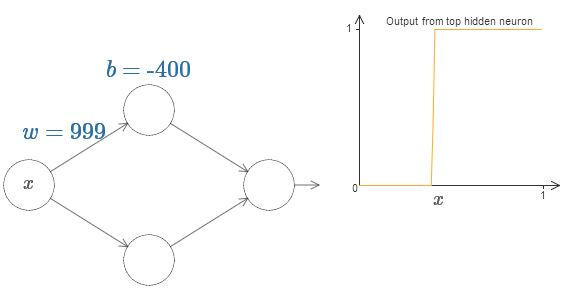# Neural Networks and Deep Learning（四）图解神经网络为什么能拟合任意函数

1. 并不是说神经网络可以精确计算任意一个函数$f(x)$，而是说当隐藏层神经元增加时，可以无限逼近$f(x)$，比如对于任何一个输入$x$，网络的输出$g(x)$和正确值$f(x)$的差小于某个阈值，$|g(x) - f(x)| < \epsilon$
2. 神经网络拟合的是连续函数，而不是那种不连续、离散、急剧变化的函数。